$$\newcommand{\id}{\mathrm{id}}$$ $$\newcommand{\Span}{\mathrm{span}}$$ $$\newcommand{\kernel}{\mathrm{null}\,}$$ $$\newcommand{\range}{\mathrm{range}\,}$$ $$\newcommand{\RealPart}{\mathrm{Re}}$$ $$\newcommand{\ImaginaryPart}{\mathrm{Im}}$$ $$\newcommand{\Argument}{\mathrm{Arg}}$$ $$\newcommand{\norm}{\| #1 \|}$$ $$\newcommand{\inner}{\langle #1, #2 \rangle}$$ $$\newcommand{\Span}{\mathrm{span}}$$

4.1: Algebra of Functions

$$\newcommand{\vecs}{\overset { \rightharpoonup} {\mathbf{#1}} }$$

$$\newcommand{\vecd}{\overset{-\!-\!\rightharpoonup}{\vphantom{a}\smash {#1}}}$$

Skills to develop

• Identify and evaluate polynomial functions.
• Multiply and divide functions.

Polynomial Functions

Any polynomial with one variable is a function and can be written in the form

$$f(x)=a_nx^n+a_{n−1}x^{n−1} + ⋯ +a_1x + a_0.$$

Here $$a_{n}$$ represents any real number and $$n$$ represents any whole number. The degree of a polynomial with one variable is the largest exponent of all the terms. Typically, we arrange terms of polynomials in descending order based on their degree and classify them as follows:

$$\begin{array} { l r } { f ( x ) = 2 } & { \color{Cerulean} { Constant\: function\: (degree }\: 0 ) } \\ { g ( x ) = 3 x + 2 } & { \color{Cerulean} { Linear\: function\: (degree\: } 1 ) } \\ { h ( x ) = 4 x ^ { 2 } + 3 x + 2 } & { \color{Cerulean} { Quadratic\: function\: (degree\: } 2 ) } \\ { r ( x ) = 5 x ^ { 3 } + 4 x ^ { 2 } + 3 x + 2}&{ \color{Cerulean} { Cubic\: function\: (degree\: } 3 ) } \end{array}$$

In this textbook, we call any polynomial with degree higher than $$3$$ an $$n$$th-degree polynomial. For example, if the degree is $$4$$, we call it a fourth-degree polynomial; if the degree is $$5$$, we call it a fifth-degree polynomial, and so on.

Example $$\PageIndex{1}$$:

Given $$f(x)=x^{2}−8x+17$$, find $$f(2)$$ and $$f(4)$$.

Solution:

Replace each instance of $$x$$ with the value given inside the parentheses.

 \begin{aligned} f ( \color{Cerulean}{2}\color{black}{ )} & = ( \color{Cerulean}{2}\color{black}{ )} ^ { 2 } - 8 ( \color{Cerulean}{2}\color{black}{ )} + 17 \\ & = 4 - 16 + 17 \\ & = 4 + 1 \\ & = 5 \end{aligned} \begin{aligned} f ( \color{Cerulean}{4}\color{black}{ ) }& = ( \color{Cerulean}{4}\color{black}{ )} ^ { 2 } - 8 ( \color{Cerulean}{4}\color{black}{ )} + 17 \\ & = 16 - 32 + 17 \\ & = - 16 + 17 \\ & = 1 \end{aligned}

Table 4.1.1

We can write $$f(2)=5$$ and $$f(4)=1$$. Remember that $$f(x)=y$$ and so we can interpret these results on the graph as follows: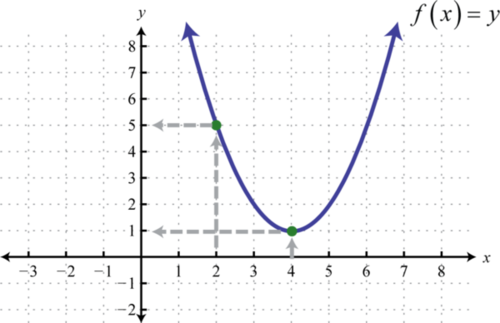Table 4.1.1

$$f(2)=5; f(4)=1$$

Often we will be asked to evaluate polynomials for algebraic expressions.

Example $$\PageIndex{2}$$:

Given $$g(x)=x^{3}−x+5$$, find $$g(−2u)$$ and $$g(x−2)$$.

Solution:

Replace $$x$$ with the expressions given inside the parentheses.

 \begin{aligned} g (\color{Cerulean}{ - 2 u} \color{black}{) }& = (\color{Cerulean}{ - 2 u}\color{black}{ )} ^ { 3 } - ( \color{Cerulean}{- 2 u}\color{black}{ )} + 5 \\ & = - 8 u ^ { 3 } + 2 u + 5 \end{aligned} \begin{aligned} g ( \color{Cerulean}{x - 2}\color{black}{ )} & = ( \color{Cerulean}{x - 2}\color{black}{ )} ^ { 3 } - (\color{Cerulean}{ x - 2}\color{black}{)} +5 \\ & = ( x - 2 ) (x-2)(x-2) - ( x -2) + 5 \\ & = ( x - 2 ) ( x^{2} - 4x +4 ) -x +7 \\ & = x ^ { 3 } - 4 x ^ { 2 } + 4 x - 2x^{2} + 8x -8 -x +7 \\ & = x ^ { 3 } - 6 x ^ { 2 } + 11x - 1 \end{aligned}

Table 4.1.2

$$g(−2u)=−8u^{2}+2u+5$$ and $$g(x−2)=x^{3}−6x^{2}+11x−1$$

The height of an object launched upward, ignoring the effects of air resistance, can be modeled with the following quadratic function:

$$h(t)=−\frac{1}{2}gt^2+v_{0}t+s_{0}$$

With this formula, the height $$h(t)$$ can be calculated at any given time $$t$$ after the object is launched. The letter $$g$$ represents acceleration due to gravity on the surface of the Earth, which is $$32$$ feet per second squared (or, using metric units, $$g = 9.8$$ meters per second squared). The variable $$v_{0}$$, pronounced “v-naught,” or sometimes “v-zero,” represents the initial velocity of the object, and $$s_{0}$$ represents the initial height from which the object was launched.

Example $$\PageIndex{3}$$

An object is launched from the ground at a speed of $$64$$ feet per second. Write a function that models the height of the object and use it to calculate the objects height at $$1$$ second and at $$3.5$$ seconds.

Solution:

We know that the acceleration due to gravity is $$g = 32$$ feet per second squared and we are given the initial velocity $$v_{0}=64$$ feet per second. Since the object is launched from the ground, the initial height is $$s_{0}=0$$ feet. Create the mathematical model by substituting these coefficients into the following formula:

$$\begin{array} { l } { h ( t ) = - \frac { 1 } { 2 } g t ^ { 2 } + v _ { 0 } t + s _ { 0 } } \\ { h ( t ) = - \frac { 1 } { 2 } ( \color{Cerulean}{32}\color{black}{ )} t ^ { 2 } + ( \color{Cerulean}{64}\color{black}{ )} t + \color{Cerulean}{0} } \\ { h ( t ) = - 16 t ^ { 2 } + 64 t } \end{array}$$

Use this model to calculate the height of the object at $$1$$ second and $$3.5$$ seconds.

$$\begin{array} { l } { h ( \color{Cerulean}{1}\color{black}{ )} = - 16 ( \color{Cerulean}{1}\color{black}{ )} ^ { 2 } + 64 ( \color{Cerulean}{1}\color{black}{ )} = - 16 + 64 = 48 } \\ { h ( \color{Cerulean}{3.5}\color{black}{ )} = - 16 ( \color{Cerulean}{3.5}\color{black}{ )} ^ { 2 } + 64 ( \color{Cerulean}{3.5}\color{black}{ )} = - 196 + 224 = 28 } \end{array}$$

$$h ( t ) = - 16 t ^ { 2 } + 64 t$$; At $$1$$ second the object is at a height of $$48$$ feet, and at $$3.5$$ seconds it is at a height of $$28$$ feet.

Exercise $$\PageIndex{1}$$

An object is dropped from a height of 6 meters. Write a function that models the height of the object and use it to calculate the object’s height $$1$$ second after it is dropped.

$$h ( t ) = - 4.9 t ^ { 2 } + 6$$; At $$1$$ second the object is at a height of $$1.1$$ meters.

The notation used to indicate addition1 and subtraction2 of functions follows:

Addition of functions: $$(f+g)(x)=f(x)+g(x)$$

Subtraction of functions: $$(f−g)(x)=f(x)−g(x)$$

When using function notation, be careful to group the entire function and add or subtract accordingly.

Example $$\PageIndex{4}$$

Given $$f(x)=x^{3}−5x−7$$ and $$g(x)=3x^{2}+7x−2$$, find $$(f+g)(x)$$ and $$(f−g)(x)$$.

Solution:

The notation $$f+g$$ indicates that we should add the given expressions.

\begin{aligned} ( f + g ) ( x ) & = f ( x ) + g ( x ) \\ & = \left( x ^ { 3 } - 5 x - 7 \right) + \left( 3 x ^ { 2 } + 7 x - 2 \right) \\ & = x ^ { 3 } - 5 x - 7 + 3 x ^ { 2 } + 7 x - 2 \\ & = x ^ { 3 } + 3 x ^ { 2 } + 2 x - 9 \end{aligned}

The notation $$f−g$$ indicates that we should subtract the given expressions. When subtracting, the parentheses become very important. Recall that we can eliminate them after applying the distributive property.

\begin{aligned} ( f - g ) ( x ) & = f ( x ) - g ( x ) \\ & = \left( x ^ { 3 } - 5 x - 7 \right) - \left( 3 x ^ { 2 } + 7 x - 2 \right) \\ & = x ^ { 3 } - 5 x - 7 - 3 x ^ { 2 } - 7 x + 2 \\ & = x ^ { 3 } - 3 x ^ { 2 } - 12 x - 5 \end{aligned}

$$(f+g)(x)=x^{3}+3x^{2}+2x−9$$ and $$(f−g)(x)=x^{3}−3x^{2}−12x−5$$

We may be asked to evaluate the sum or difference of two functions. We have the option to first find the sum or difference in general and then use the resulting function to evaluate for the given variable, or evaluate each first and then find the sum or difference.

Example $$\PageIndex{5}$$

Evaluate $$(f−g)(3)$$ given $$f(x)=5x^{2}−x+4$$ and $$g(x)=x^{2}+2x−3$$.

Solution:

First, find $$(f−g)(x)$$.

\begin{aligned} ( f - g ) ( x ) & = f ( x ) - g ( x ) \\ & = \left( 5 x ^ { 2 } - x + 4 \right) - \left( x ^ { 2 } + 2 x - 3 \right) \\ & = 5 x ^ { 2 } - x + 4 - x ^ { 2 } - 2 x + 3 \\ & = 4 x ^ { 2 } - 3 x + 7 \end{aligned}

Therefore,

$$( f - g ) ( x ) = 4 x ^ { 2 } - 3 x + 7$$.

Next, substitute $$3$$ in for the variable $$x$$.

\begin{aligned} ( f - g ) ( \color{OliveGreen}{3}\color{black}{ )} & = 4 ( \color{OliveGreen}{3}\color{black}{ )} ^ { 2 } - 3 (\color{OliveGreen}{ 3}\color{black}{ )} + 7 \\ & = 36 - 9 + 7 \\ & = 34 \end{aligned}

Hence $$(f−g)(3)=34$$.

Alternate Solution

Since $$(f−g)(3)=f(3)−g(3)$$, we can find $$f(3)$$ and $$g(3)$$ and then subtract the results.

 \begin{aligned} f ( x ) & = 5 x ^ { 2 } - x + 4 \\ f ( \color{Cerulean}{3}\color{black}{ )} & = 5 ( \color{Cerulean}{3}\color{black}{ )} ^ { 2 } - ( \color{Cerulean}{3}\color{black}{ )} + 4 \\ & = 45 - 3 + 4 \\ & = 46 \end{aligned} \begin{aligned} g ( x ) & = x ^ { 2 } + 2 x - 3 \\ g ( \color{Cerulean}{3}\color{black}{ )} & = (\color{Cerulean}{ 3}\color{black}{ )} ^ { 2 } + 2 ( \color{Cerulean}{3}\color{black}{ )} - 3 \\ & = 9 + 6 - 3 \\ & = 12 \end{aligned}

Table 4.1.3

Therefore,

\begin{aligned} ( f - g ) ( 3 ) & = f ( 3 ) - g ( 3 ) \\ & = 46 - 12 \\ & = 34 \end{aligned}

Notice that we obtain the same answer.

$$(f−g)(3)=34$$

Note

If multiple values are to be evaluated, it is best to find the sum or difference in general first and then use it to evaluate.

Exercise $$\PageIndex{2}$$

Evaluate $$(f+g)(−1)$$ given $$f(x)=x^{3}+x−8$$ and $$g(x)=2x^{2}−x+9$$.

$$2$$

Multiplying and Dividing Functions

The notation used to indicate multiplication3 and division4 of functions follows:

Multiplication of functions: $$( f \cdot g ) ( x ) = f ( x ) \cdot g ( x )$$ $$( f / g ) ( x ) = \frac { f ( x ) } { g ( x ) } , \text { where } g ( x ) \neq 0$$

Table 4.1.4

Example $$\PageIndex{6}$$:

Given $$f(x)=15x^{4}−9x^{3}+6x^{2}$$ and $$g(x)=3x^{2}$$, find $$(f⋅g)(x)$$ and $$(f/g)(x)$$.

Solution:

The notation $$f⋅g$$ indicates that we should multiply. Apply the distributive property and simplify.

\begin{aligned} ( f \cdot g ) ( x ) & = f ( x ) \cdot g ( x ) \\ & = \left( 15 x ^ { 4 } - 9 x ^ { 3 } + 6 x ^ { 2 } \right) \left( 3 x ^ { 2 } \right) \\ & = 15 x ^ { 4 } \cdot \color{Cerulean}{3 x ^ { 2 }}\color{black}{ -} 9 x ^ { 3 } \cdot \color{Cerulean}{3 x ^ { 2} }\color{black}{ +} 6 x ^ { 2 } \cdot \color{Cerulean}{3 x ^ { 2} } \\ & = 45 x ^ { 6 } - 27 x ^ { 5 } + 18 x ^ { 4 } \end{aligned}

The notation $$f /g$$ indicates that we should divide. For this quotient, assume $$x ≠ 0$$.

\begin{aligned} ( f / g ) ( x ) & = \frac { f ( x ) } { g ( x ) } \\ & = \frac { 15 x ^ { 4 } - 9 x ^ { 3 } + 6 x ^ { 2 } } { 3 x ^ { 2 } } \\ & = \frac { 15 x ^ { 4 } } { 3 x ^ { 2 } } - \frac { 9 x ^ { 3 } } { 3 x ^ { 2 } } + \frac { 6 x ^ { 2 } } { 3 x ^ { 2 } } \\ & = 5 x ^ { 2 } - 3 x + 2 \end{aligned}

$$(f⋅g)(x)=45x^{6}−27x^{5}+18x^{4}$$ and $$(f/g)(x)=5x^{2}−3x+2$$ where $$x≠0$$.

Example $$\PageIndex{7}$$:

Given $$f(x)=6x−5$$ and $$g(x)=3x^{2}−2x−1$$, evaluate $$(f⋅g)(0)$$ and $$(f⋅g)(−1)$$

Solution:

Begin by finding $$(f⋅g)(x)$$.

\begin{aligned} ( f \cdot g ) ( x ) & = f ( x ) \cdot g ( x ) \\ & = ( 6 x - 5 ) \left( 3 x ^ { 2 } - 2 x - 1 \right) \\ & = 18 x ^ { 3 } - 12 x ^ { 2 } - 6 x - 15 x ^ { 2 } + 10 x + 5 \\ & = 18 x ^ { 3 } - 27 x ^ { 2 } + 4 x + 5 \end{aligned}

Therefore $$( f \cdot g ) ( x ) = 18 x ^ { 3 } - 27 x ^ { 2 } + 4 x + 5$$, and we have,

 \begin{aligned} ( f \cdot g ) ( \color{Cerulean}{0}\color{black}{ )} & = 18 ( \color{Cerulean}{0}\color{black}{ )} ^ { 3 } - 27 ( \color{Cerulean}{0}\color{black}{ )} ^ { 2 } + 4 ( \color{Cerulean}{0}\color{black}{ )} + 5 \\ & = 5 \end{aligned} \begin{aligned} ( f \cdot g ) ( \color{Cerulean}{-1}\color{black}{ )} & = 18 ( \color{Cerulean}{-1}\color{black}{ )} ^ { 3 } - 27 ( \color{Cerulean}{-1}\color{black}{ )} ^ { 2 } + 4 ( \color{Cerulean}{-1}\color{black}{ )} + 5 \\ & = -18-27-4+5\\ &=-44 \end{aligned}

Table 4.1.5

$$( f \cdot g ) ( 0 ) = 5 \text { and } ( f \cdot g ) ( - 1 ) = - 44$$

Exercise $$\PageIndex{3}$$

Evaluate $$(f⋅g)(−1)$$ given $$f(x)=x^{3}+x−8$$ and $$g(x)=2x^{2}−x+9$$.

$$-120$$

Here we explore the geometry of adding functions. One way to do this is to use the fact that $$(f+g)(x)=f(x)+g(x)$$. Add the functions together using x-values for which both f and g are defined.

Example $$\PageIndex{8}$$

Use the graphs of $$f$$ and $$g$$ to graph $$f+g$$. Also, give the domain of $$f+g$$.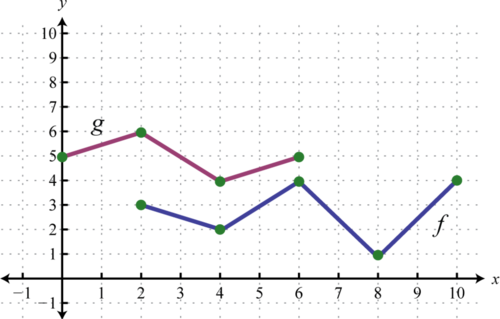Figure 4.1.2

Solution:

In this case, both functions are defined for $$x$$-values between $$2$$ and $$6$$. We will use $$2$$, $$4$$, and $$6$$ as representative values in the domain of $$f+g$$ to sketch its graph.

$$\begin{array} { l } { ( f + g ) ( \color{Cerulean}{2}\color{black}{ )} = f ( \color{Cerulean}{2}\color{black}{ )} + g (\color{Cerulean}{ 2}\color{black}{ )} = 3 + 6 = 9 } \\ { ( f + g ) ( \color{Cerulean}{4}\color{black}{ )} = f ( \color{Cerulean}{4}\color{black}{ )} + g ( \color{Cerulean}{4}\color{black}{ )} = 2 + 4 = 6 } \\ { ( f + g ) ( \color{Cerulean}{6}\color{black}{ )} = f ( \color{Cerulean}{6}\color{black}{ )} + g ( \color{Cerulean}{6}\color{black}{ )} = 4 + 5 = 9 } \end{array}$$

Sketch the graph of f+g using the three ordered pair solutions $$(2,9), (4,6)$$, and $$(6,9)$$.Figure 4.1.3

$$f+g$$ graphed above has domain $$[2,6]$$.

Example $$\PageIndex{9}$$

Use the graphs of $$f$$ and $$g$$ to graph $$f+g$$. Also, give the domain of $$f+g$$.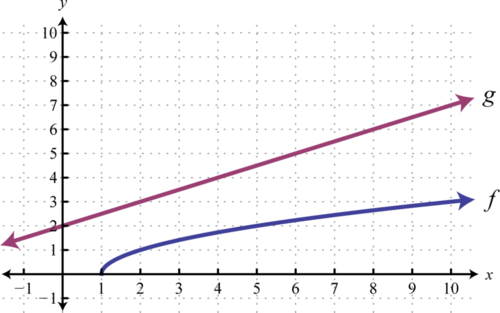Figure 4.1.4

Solution:

Another way to add nonnegative functions graphically is to copy the line segment formed from the $$x$$-axis to one of the functions onto the other as illustrated below.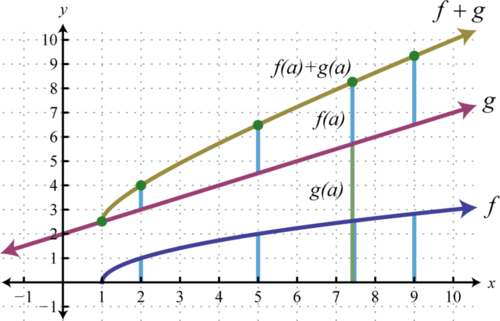Figure 4.1.5

The line segment from the $$x$$-axis to the function $$f$$ represents $$f(a)$$. Copy this line segment onto the other function over the same point; the endpoint represents $$f(a)+g(a)$$. Doing this for a number of points allows us to obtain a quick sketch of the combined graph. In this example, the domain of $$f+g$$ is limited to the $$x$$-values for which $$f$$ is defined.

Domain: $$[1,∞)$$Figure 4.1.6

In general, the domain of $$f+g$$ is the intersection of the domain of $$f$$ with the domain of $$g$$. In fact, this is the case for all of the arithmetic operations with an extra consideration for division. When dividing functions, we take extra care to remove any values that make the denominator zero. This will be discussed in more detail as we progress in algebra.

Key Takeaways

• Any polynomial with one variable is a function and can be written in the form $$f ( x ) = a _ { n } x ^ { n } + a _ { n - 1 } x ^ { n - 1 } + \ldots + a _ { 1 } x + a _ { 0 }$$. The degree of the polynomial is the largest exponent of all the terms.
• Use function notation to streamline the evaluating process. Substitute the value or expression inside the parentheses for each instance of the variable.
• The notation $$(f+g)(x)$$ indicates that we should add $$f(x)+g(x)$$.
• The notation $$(f−g)(x)$$ indicates that we should subtract $$f(x)−g(x)$$.
• The notation $$(f⋅g)(x)$$ indicates that we should multiply $$f(x)g(x)$$.
• The notation $$(f/g)(x)$$ indicates that we should divide $$\frac{f(x)}{g(x)}$$, where $$g(x)≠0$$.
• The domain of the function that results from these arithmetic operations is the intersection of the domain of each function. The domain of a quotient is further restricted to values that do not evaluate to zero in the denominator.

Exercise $$\PageIndex{4}$$

Evaluate.

1. Given $$f(x)=x^{2}−10x+3$$, find $$f(−3), f(0)$$, and $$f(5)$$.

2. Given $$f(x)=2x^{2}−x+9$$, find $$f(−1), f(0)$$, and $$f(3)$$.

3. Given $$g(x)=x^{3}−x^{2}+x+7$$, find $$g(−2), g(0)$$, and $$g(3)$$.

4. Given $$g(x)=x^{3}−2x+5$$, find $$g(−5), g(0)$$, and $$g(3)$$.

5. Given $$s(t)=5t^{4}−t^{2}+t−3$$, find $$s(−1), s(0)$$, and $$s(2)$$.

6. Given $$p(n)=n^{4}−10n^{2}+9$$, find $$p(−3), p(−1)$$, and $$p(2)$$.

7. Given $$f(x)=x^{6}−64$$, find $$f(−2), f(−1)$$, and $$f(0)$$.

8. Given $$f(x)=x^{6}−x^{3}+3$$, find $$f(−2), f(−1)$$, and $$f(0)$$.

9. Given $$f(x)=x^{2}−2x−1$$, find $$f(2t)$$ and $$f(2t−1)$$.

10. Given $$f(x)=x^{2}−2x+4$$, find $$f(−3t)$$ and $$f(2−3t)$$.

11. Given $$g(x)=2x^{2}+3x−1$$, find $$g(−5a)$$ and $$g(5−2x)$$.

12. Given $$g(x)=3x^{2}−5x+4$$, find $$g(−4u)$$ and $$g(3x−1)$$.

13. Given $$f(x)=x^{3}−1$$, find $$f(2a)$$ and $$f(x−2)$$.

14. Given $$f(x)=x^{3}−x+1$$, find $$f(−3x)$$ and $$f(2x+1)$$.

15. Given $$g(x)=x^{3}+x^{2}−1$$, find $$g(x^{2})$$ and $$g(x−4)$$.

16. Given $$g(x)=2x^{3}−x+1$$, find $$g(−2x^{3})$$ and $$g(3x−1)$$.

1. $$f ( - 3 ) = 42 ; f ( 0 ) = 3 ; f ( 5 ) = - 22$$

3. $$g ( - 2 ) = - 7 ; g ( 0 ) = 7 ; g ( 3 ) = 28$$

5. $$s ( - 1 ) = 0 ; s ( 0 ) = - 3 ; s ( 2 ) = 75$$

7. $$f ( - 2 ) = 0 ; f ( - 1 ) = - 63 ; f ( 0 ) = - 64$$

9. $$f ( 2 t ) = 4 t ^ { 2 } - 4 t - 1 : f ( 2 t - 1 ) = 4 t ^ { 2 } - 8 t + 2$$

11. $$g ( - 5 a ) = 50 a ^ { 2 } - 15 a - 1 ; g ( 5 - 2 x ) = 8 x ^ { 2 } - 46 x + 64$$

13. $$f ( 2 a ) = 8 a ^ { 3 } - 1 ; f ( x - 2 ) = x ^ { 3 } - 6 x ^ { 2 } + 12 x - 9$$

15. $$g \left( x ^ { 2 } \right) = x ^ { 6 } + x ^ { 4 } - 1 ; g ( x - 4 ) = x ^ { 3 } - 11 x ^ { 2 } + 40 x - 49$$

Exercise $$\PageIndex{5}$$

Given the function calculate $$f(x+h)$$.

1. $$f(x)=5x−3$$

2. $$f(x)=x^{2}−1$$

3. $$f(x)=x^{3}−8$$

4. $$f(x)=x^{4}$$

1. $$f ( x + h ) = 5 x + 5 h - 3$$

3. $$f ( x + h ) = x ^ { 3 } + 3 h x ^ { 2 } + 3 h ^ { 2 } x + h ^ { 3 } - 8$$

Exercise $$\PageIndex{6}$$

Given the graph of the polynomial function $$f$$ find the function values.

1. Find $$f(0), f(1)$$, and $$f(2)$$.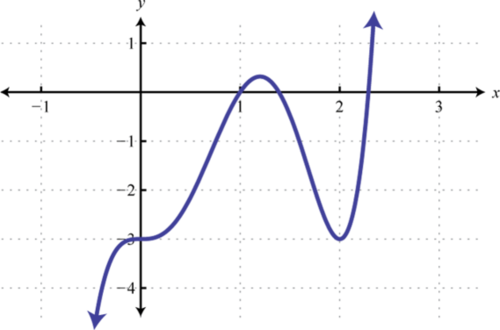Figure 4.1.7

2. Find $$f(−1), f(0)$$, and $$f(1)$$.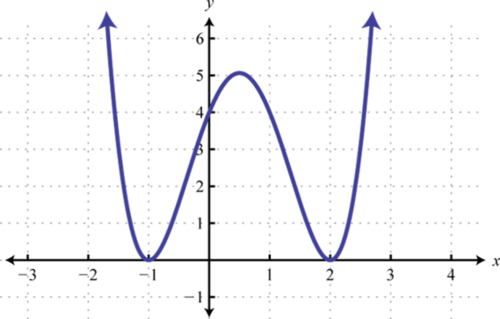Figure 4.1.8

3. Find $$f(−2), f(−1)$$, and $$f(0)$$.Figure 4.1.9

4. Find $$f(−3), f(−2)$$, and $$f(0)$$.Figure 4.1.10

5. A projectile is launched upward from the ground at a speed of $$48$$ feet per second. Write a function that models the height of the projectile and use it to calculate the height every $$1/2$$ second after launch. Sketch a graph that shows the height of the projectile with respect to time.

6. An object is tossed upward from a $$48$$-foot platform at a speed of $$32$$ feet per second. Write a function that models the height of the object and use it to calculate the height every $$1/2$$ second after the object is tossed. Sketch a graph that shows the height of the object with respect to time.

7. An object is dropped from a $$128$$-foot bridge. Write a function that models the height of the object, and use it to calculate the height at $$1$$ second and $$2$$ seconds after it has been dropped.

8. An object is dropped from a $$500$$-foot building. Write a function that models the height of the object, and use it to calculate the distance the object falls in the $$1$$st second, $$2$$nd second, and the $$3$$rd second.

9. A bullet is fired straight up into the air at $$320$$ meters per second. Ignoring the effects of air friction, write a function that models the height of the bullet, and use it to calculate the bullet’s height $$1$$ minute after it was fired into the air.

10. A book is dropped from a height of $$10$$ meters. Write a function that gives the height of the book, and use it to determine how far it will fall in $$1 \frac{1}{4}$$ seconds.

1. $$f ( 0 ) = - 3 ; f ( 1 ) = 0 ; f ( 2 ) = - 3$$

3. $$f ( - 2 ) = 2 ; f ( - 1 ) = - 7 ; f ( 0 ) = - 2$$

5. $$h ( t ) = - 16 t ^ { 2 } + 48 t$$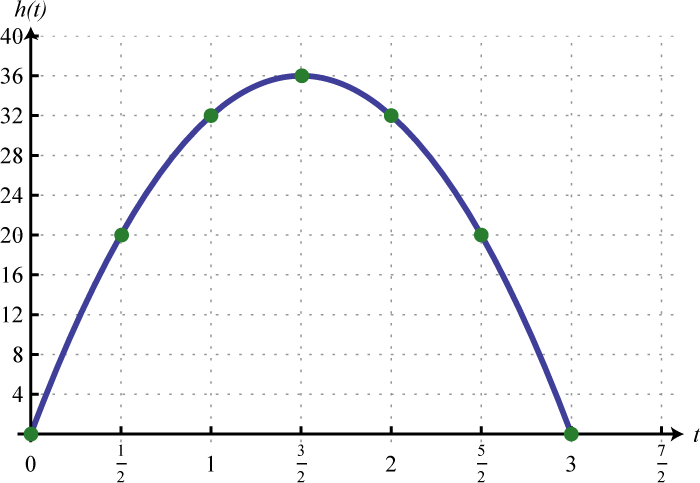Figure 4.1.11

7. $$h ( t ) = - 16 t ^ { 2 } + 128$$; At $$1$$ second the object’s height is $$112$$ feet and at $$2$$ seconds its height is $$64$$ feet.

9. $$h ( t ) = - 4.9 t ^ { 2 } + 320 t ; 1,560$$ meters

Exercise $$\PageIndex{7}$$

Given functions $$f$$ and $$g$$, find $$(f+g)$$ and $$(f-g)$$.

1. $$f(x)=5x−3, g(x)=4x−1$$

2. $$f(x)=3x+2, g(x)=7x−5$$

3. $$f(x)=2−3x, g(x)=1−x$$

4. $$f(x)=8x−5, g(x)=−7x+4$$

5. $$f(x)=x^{2}−3x+2, g(x)=x^{2}+4x−7$$

6. $$f(x)=2x^{2}+x−3, g(x)=x^{2}−x+4$$

7. $$f(x)=x^{2}+5x−3, g(x)=6x+11$$

8. $$f(x)=9x+5, g(x)=2x^{2}−5x+4$$

9. $$f(x)=9x^{2}−1, g(x)=x^{2}+5x$$

10. $$f(x)=10x^{2}, g(x)=5x^{2}−8$$

11. $$f(x)=8x^{3}+x−4, g(x)=4x^{3}+x^{2}−1$$

12. $$f(x)=x^{3}−x^{2}+x+1, g(x)=x^{3}−x^{2}−x−1$$

1. $$( f + g ) ( x ) = 9 x - 4 ; ( f - g ) ( x ) = x - 2$$

3. $$( f + g ) ( x ) = - 4 x + 3 ; ( f - g ) ( x ) = - 2 x + 1$$

5. $$( f + g ) ( x ) = 2 x ^ { 2 } + x - 5 ; ( f - g ) ( x ) = - 7 x + 9$$

7. $$( f + g ) ( x ) = x ^ { 2 } + 11 x + 8 ; ( f - g ) ( x ) = x ^ { 2 } - x - 14$$

9. $$( f + g ) ( x ) = 10 x ^ { 2 } + 5 x - 1 ; ( f - g ) ( x ) = 8 x ^ { 2 } - 5 x - 1$$

11. $$\begin{array} { l } { ( f + g ) ( x ) = 12 x ^ { 3 } + x ^ { 2 } + x - 5 } \\ { ( f - g ) ( x ) = 4 x ^ { 3 } - x ^ { 2 } + x - 3 } \end{array}$$

Exercise $$\PageIndex{8}$$

Given $$f ( x ) = x ^ { 3 } + 2 x ^ { 2 } - 8$$ and $$g ( x ) = 2 x ^ { 2 } - 3 x + 5$$, evaluate the following.

1. $$(f+g)(−2)$$

2. $$(f+g)(3)$$

3. $$(f−g)(−2)$$

4. $$(f−g)(3)$$

5. $$(g−f)(−2)$$

6. $$(g−f)(3)$$

7. $$(f+f)(1)$$

8. $$(g+g)(−1)$$

1. $$11$$

3. $$-27$$

5. $$27$$

7. $$-10$$

Exercise $$\PageIndex{9}$$

Given the graphs of $$f$$ and $$g$$, evaluate the following.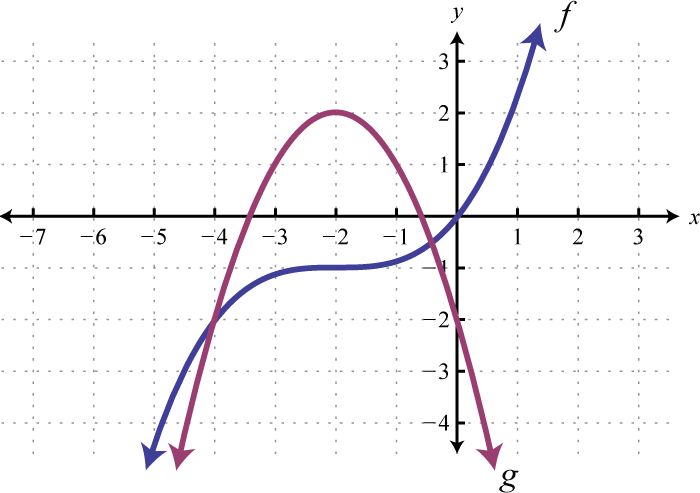Figure 4.1.12

1. $$(f+g)(−4)$$

2. $$(f−g)(−4)$$

3. $$(f+g)(−2)$$

4. $$(f−g)(−2)$$

5. $$(f+g)(0)$$

6. $$(f−g)(0)$$

1. $$-4$$

3. $$1$$

5. $$-2$$

Exercise $$\PageIndex{10}$$

Given $$f$$ and $$g$$, find $$f⋅g$$.

1. $$f(x)=5x, g(x)=x−3$$

2. $$f(x)=x−4, g(x)=6x$$

3. $$f(x)=2x−3, g(x)=3x+4$$

4. $$f(x)=5x−1, g(x)=2x+1$$

5. $$f(x)=3x+4, g(x)=3x−4$$

6. $$f(x)=x+5, g(x)=x−5$$

7. $$f(x)=x−2, g(x)=x^{2}−3x+2$$

8. $$f(x)=2x−3, g(x)=x^{2}+2x−1$$

9. $$f(x)=2x^{2}, g(x)=x^{2}−7x+5$$

10. $$f(x)=5x^{3}, g(x)=x^{2}−3x−1$$

11. $$f(x)=x^{2}−3x−2, g(x)=2x^{2}−x+3$$

12. $$f(x)=x^{2}+x−1, g(x)=x^{2}−x+1$$

1. $$( f \cdot g ) ( x ) = 5 x ^ { 2 } - 15 x$$

3. $$( f \cdot g ) ( x ) = 6 x ^ { 2 } - x - 12$$

5. $$( f \cdot g ) ( x ) = 9 x ^ { 2 } - 16$$

7. $$( f \cdot g ) ( x ) = x ^ { 3 } - 5 x ^ { 2 } + 8 x - 4$$

9. $$( f \cdot g ) ( x ) = 2 x ^ { 4 } - 14 x ^ { 3 } + 10 x ^ { 2 }$$

11. $$( f \cdot g ) ( x ) = 2 x ^ { 4 } - 7 x ^ { 3 } + 2 x ^ { 2 } - 7 x - 6$$

Exercise $$\PageIndex{11}$$

Given $$f$$ and $$g$$, find $$f/g$$. (Assume all expressions in the denominator are nonzero.)

1. $$f ( x ) = 36 x ^ { 3 } - 16 x ^ { 2 } - 8 x , g ( x ) = 4 x$$
2. $$f ( x ) = 2 x ^ { 3 } - 6 x ^ { 2 } + 10 x , g ( x ) = 2 x$$
3. $$f ( x ) = 20 x ^ { 7 } - 15 x ^ { 5 } + 5 x ^ { 3 } , g ( x ) = 5 x ^ { 3 }$$
4. $$f ( x ) = 9 x ^ { 6 } + 12 x ^ { 4 } - 3 x ^ { 2 } , g ( x ) = 3 x ^ { 2 }$$
5. $$f ( x ) = x ^ { 3 } + 4 x ^ { 2 } + 3 x - 2 , g ( x ) = x + 2$$
6. $$f ( x ) = x ^ { 3 } - x ^ { 2 } - 10 x + 12 , g ( x ) = x - 3$$
7. $$f ( x ) = 6 x ^ { 3 } - 13 x ^ { 2 } + 36 x - 45 , g ( x ) = 2 x - 3$$
8. $$f ( x ) = 6 x ^ { 3 } - 11 x ^ { 2 } + 15 x - 4 , g ( x ) = 3 x - 1$$
9. $$f ( x ) = 3 x ^ { 3 } - 13 x ^ { 2 } - x + 8 , g ( x ) = 3 x + 2$$
10. $$f ( x ) = 5 x ^ { 3 } - 16 x ^ { 2 } + 13 x - 6 , g ( x ) = 5 x - 1$$

1. $$( f / g ) ( x ) = 9 x ^ { 2 } - 4 x - 2$$

3. $$( f / g ) ( x ) = 4 x ^ { 4 } - 3 x ^ { 2 } + 1$$

5. $$( f / g ) ( x ) = x ^ { 2 } + 2 x - 1$$

7. $$( f / g ) ( x ) = 3 x ^ { 2 } - 2 x + 15$$

9. $$( f / g ) ( x ) = x ^ { 2 } - 5 x + 3 + \frac { 2 } { 3 x + 2 }$$

Exercise $$\PageIndex{12}$$

Given $$f ( x ) = 25 x ^ { 4 } + 10 x ^ { 3 } - 5 x ^ { 2 }$$ and $$g(x) = 5x^{2}$$ evaluate the following.

1. $$(f⋅g)(−1)$$

2. $$(f⋅g)(1)$$

3. $$(f/g)(−2)$$

4. $$(f/g)(−3)$$

5. $$(g⋅f)(0)$$

6. $$(g/f)(1)$$

7. $$(g⋅g)(−1)$$

8. $$(f⋅f)(−1)$$

1. $$50$$

3. $$15$$

5. $$0$$

7. $$25$$

Exercise $$\PageIndex{13}$$

Given the graphs of $$f$$ and $$g$$ evaluate the following.Figure 4.1.13

1. $$(f⋅g)(3)$$

2. $$(f⋅g)(5)$$

3. $$(f/g)(5)$$

4. $$(f/g)(3)$$

5. $$(f⋅g)(1)$$

6. $$(f/g)(1)$$

1. $$-2$$

3. $$0$$

5. $$4$$

Exercise $$\PageIndex{14}$$

Given $$f ( x ) = 5 x ^ { 3 } - 15 x ^ { 2 } + 10 x , g ( x ) = x ^ { 2 } - x + 3$$, and $$h(x)=-5x$$, find the following. (Assume all expressions in the denominator are nonzero.)

1. $$(f−g)(x)$$

2. $$(g−f)(x)$$

3. $$(g⋅h)(x)$$

4. $$(f/h)(x)$$

5. $$(h+g)(x)$$

6. $$(h⋅f)(x)$$

7. $$(g/h)(2)$$

8. $$(g−h)(−3)$$

9. The revenue in dollars from selling MP3 players is given by the function $$R(n)=125n−0.15n^{2}$$, where $$n$$ represents the number of units sold $$(0≤n<833)$$. The cost in dollars of producing the MP3 players is given by the formula $$C(n)=1200+42n$$ where $$n$$ represents the number of units produced. Write a function that models the profit of producing and selling $$n$$ MP3 players. Use the function to determine the profit generated from producing and selling $$225$$ MP3 players. Recall that profit equals revenues less costs.

10. The inner radius of a washer is $$\frac{1}{2}$$ that of the outer radius.Figure 4.1.14

1. $$( f - g ) ( x ) = 5 x ^ { 3 } - 16 x ^ { 2 } + 11 x - 3$$

3. $$( g \cdot h ) ( x ) = - 5 x ^ { 3 } + 5 x ^ { 2 } - 15 x$$

5. $$( h + g ) ( x ) = x ^ { 2 } - 6 x + 3$$

7. $$( g / h ) ( 2 ) = - \frac { 1 } { 2 }$$

9. $$P ( n ) = - 0.15 n ^ { 2 } + 83 n - 1200 ; \ 9,881.25$$

Exercise $$\PageIndex{15}$$

Use the graphs of $$f$$ and $$g$$ to graph $$f+g$$. Also, give the domain of $$f+g$$.

1.Figure 4.1.15

2.Figure 4.1.16

3.Figure 4.1.17

4.Figure 4.1.18

5.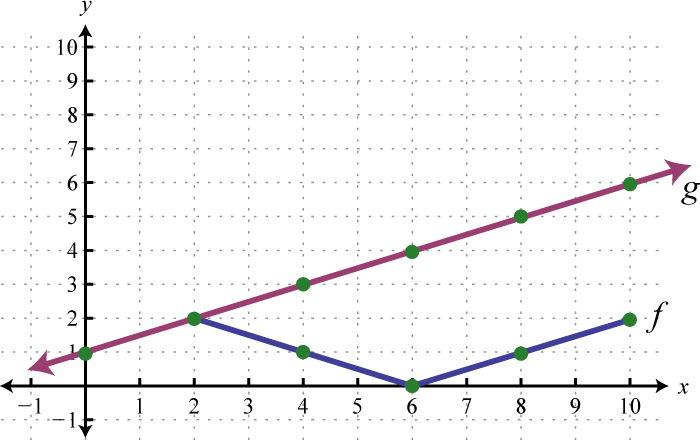Figure 4.1.19

6.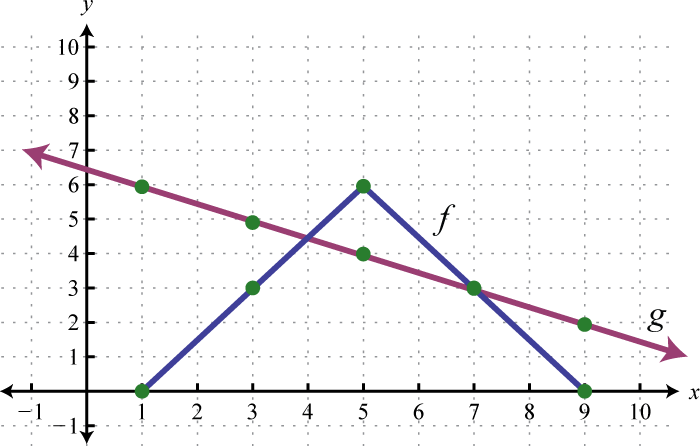Figure 4.1.20

7.Figure 4.1.21

8.Figure 4.1.22

9.Figure 4.1.23

10.Figure 4.1.24

1. $$[2,8]$$Figure 4.1.25

3. $$[0,10]$$Figure 4.1.26

5. $$[2,10]$$Figure 4.1.27

7. $$[ - 2 , \infty )$$Figure 4.1.28

9. $$( - \infty , \infty )$$Figure 4.1.29

Exercise $$\PageIndex{16}$$

1. Which arithmetic operations on functions are commutative? Explain.

2. Explore ways we can add functions graphically if they happen to be negative.

1Add functions as indicated by the notation: $$(f + g) (x) = f (x) + g (x)$$.
2Subtract functions as indicated by the notation: $$(f − g) (x) = f (x) − g (x)$$.
3Multiply functions as indicated by the notation: $$(f ⋅ g) (x) = f (x) ⋅ g (x)$$.
4Divide functions as indicated by the notation: $$(f /g) (x) = \frac{f(x)}{ g(x)}$$ , where $$g (x) ≠ 0$$.